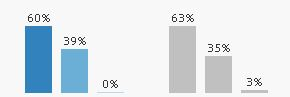New to QlikView

Discussion board where members can get started with QlikView.

Announcements
Highlighted
Contributor III

Calculating Relative % values for each X axis values

I have my Primary Dimension as Year and Product Category as my Secondary Dimension.

While calculating Sales %, I usually use Sum(Sales) with Relative option checked. If I do that, I get something like the below chartHere, the expression takes into account the values of all the 6 bars and then calculate a value. However, I need to get the relative split for each year. Something like thisThe above image has 2 Charts, one for 2014 and 2015. Is there a way to do it in a single chart?

Note: I don't want to use two expression in a single chart, because I want to make use of the Dimension selection option that is available by default and I have used this expression as Sum({1}Sales) since I have to highlight the selected Bar Chart and gray out the non selected something like thisEven though these are all work around's for a particular problem. I'm curious enough to know Is it possible to implement all the above features in a single bar chart?

Tags (1)
1 Solution

Accepted Solutions
New Contributor III

Re: Calculating Relative % values for each X axis values

Hi,

In expression, just give sum(Sales)/sum(TOTAL<year>Sales.

Thanks,

Vignesh U.

5 Replies
Esteemed Contributor III

Re: Calculating Relative % values for each X axis values

did u chkd option for all expressions?

relative

Contributor III

Re: Calculating Relative % values for each X axis values

I just have a single expression. The three bars represent Secondary Dimension values. And, Yes. I checked the relative optionNot applicable

Re: Calculating Relative % values for each X axis values

Try with

sum(Sales)/sum(Total <Year> Sales)

It should give you percentages per year

New Contributor III

Re: Calculating Relative % values for each X axis values

Hi,

In expression, just give sum(Sales)/sum(TOTAL<year>Sales.

Thanks,

Vignesh U.

New Contributor III

Re: Calculating Relative % values for each X axis values

And dont check the Relative checkbox in expression.